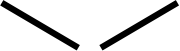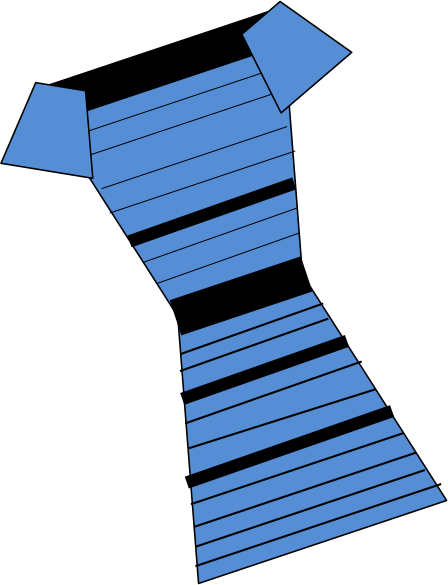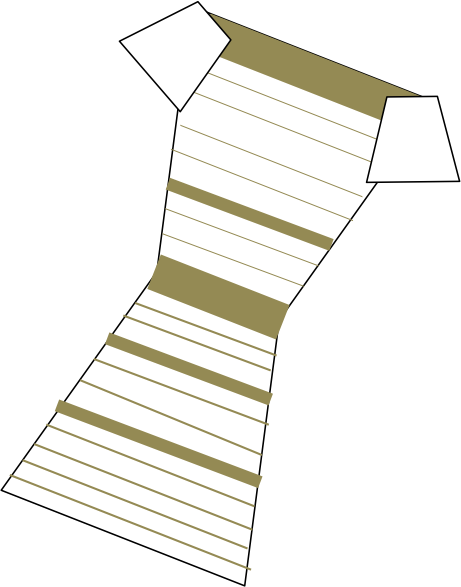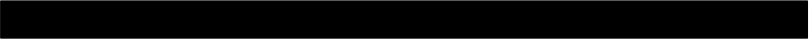# Bayesian Modeling of Vision: The Dress000

Time with blue light:

000

Time with yellow light:0
blue dresses0
gold dressesp(black and blue) means the probability that a random dress will be black and blue. The man will estimate this probability based on his past experience with dresses. That is, he will estimate p(black and blue) as the proportion of all dresses he's seen that have been black and blue, which is calculated as the number of black and blue dresses he's seen divided by the total number of dresses he's seen.
p(white and gold) means the probability that a random dress will be white and gold. The man will estimate this probability based on his past experience with dresses. That is, he will estimate p(white and gold) as the proportion of all dresses he's seen that have been white and gold, which is calculated as the number of white and gold dresses he's seen divided by the total number of dresses he's seen.
p(image | black and blue) means the probability of obtaining this specific image given that the dress in the image is black and blue. In other words, if we assume that the dress is black and blue, how likely is it that an image of it will look like this? To figure this out, we need to determine what conditions would lead to this image arising from a black and blue dress. In our scenario, the condition that leads to this is having yellow lighting when the picture was taken, because yellow lighting is what would make a black and blue dress look the way it does in the image. Therefore, in this specific scenario, we can interpret p(image | black and blue) as being the probability that the lighting when the image was taken was yellow. The man will estimate this probability based on his past experience with lighting; he'll assume that the probability of yellow lighting is the percent of his time that he has spent in yellow light, which you can calculate by taking the time he's spent in yellow light divided by the total time the simulation ran.
p(image | white and gold) means the probability of obtaining this specific image given that the dress in the image is white and gold. In other words, if we assume that the dress is white and gold, how likely is it that an image of it will look like this? To figure this out, we need to determine what conditions would lead to this image arising from a white and gold dress. In our scenario, the condition that leads to this is having blue lighting when the picture is taken, because blue lighting is what would make a white and gold dress look the way it does in the image. Therefore, in this specific scenario, we can interpret p(image | white and gold) as being the probability that the lighting when the image was taken was blue. The man will estimate this probability based on his past experience with lighting; he'll assume that the probability of blue lighting is the percent of his time that he has spent in blue light, which you can calculate by taking the time he's spent in blue light divided by the total time the simulation ran.
p(image) is the trickiest entity to calculate here. To compute it, we have to sum over all possible relevant scenarios. In this case, there are two relevant scenarios: Either the dress is black and blue, or the dress is white and gold. Since we're focused on the image here, we can further specify that the two scenarios are "we got this image, and the dress is black and blue" and "we got this image, and the dress is white and gold." Based on this, we can write p(image) = p(image, black and blue) + p(image, white and gold) (since that equation sums over our two possible scenarios). Now we can use the equations for conditional probability to rewrite this equation as p(image) = p(image | black and blue) * p(black and blue) + p(image | white and gold) * p(white and gold). Now all 4 expressions in the right hand side are things you've calculated above, so you can plug in those values you've calculated to get p(image).

Now that you’ve tried out the controls, we’re going to actually give the man the required experience. To do this, you will run a simulation for 20 seconds, during which time you can freely alter the man’s lighting and show him as many dresses of each color as you wish. Click “Start Simulation” to get started.

Now, at last, we can show the man the picture! Click "Show Picture" to do this.

But is the man seeing the picture as black and blue or as white and gold? Let's figure it out! The man will see the dress as whichever color he internally deems to be more probable, based on the way the dress looks in the image. Therefore, we will need to calculate the relevant probabilities. Which of the following entities represent the probability that the dress in the image is black and blue or white and gold, based on the way it looks in the image?

Not quite! p(black and blue) simply means the probability that a dress will be black and blue; that is, given some random dress in the world, what is the probability that the dress is black and blue? (And p(white and gold) is analogous). However, we don't want the probability that any dress will be black and blue but rather the probability that the dress in the image is black and blue.Thus, the expression we choose had better refer to the image in some way.
Not quite! p(image | black and blue) means the probability of getting an image that looks like this, given that the dress in the image is black and blue. However, we don't know what color the dress actually is, so we can't take the dress's color as a given (which is what these expressions do).
Correct! p(black and blue | image) means the probability that the dress in the image is black and blue, given that the image looks the way it does. This is exactly the probability we are trying to determine, since we know the way the image looks and want to determine how likely it is that the dress is black and blue based on that image.
Not quite! p(black and blue, image) means the probability that two things are true: The dress in the image is black and blue, and the image that you are seeing looks the way this image does. This expression basically treats both of these events as uncertainties whose probabilities have to be considered. However, this is not the case; we know that the image you are seeing looks the way this image does, so we shouldn't be treating the image's appearance as part of our uncertainty about this scenario. Instead, we want an expression that treats the fact that the image looks this way as a given.

Now we know what we need to calculate: p(black and blue | image) and p(white and gold | image). But how do we calculate it? It seems difficult to calculate these probabilities given the man's experience, since these probabilities are conditioned on the image, yet the man has never seen this image before.

The solution is to use a very useful and versatile formula called Bayes' Theorem, written below. Hover over each term in the equation for a description of what that term means:

p(A|B)

=

p(B|A)

p(A)

p(B)The posterior probability: The probability that A is true, based on some knowledge or observation B. It is called the "posterior" probability because posterior means later or after, and it is the probability of A after you have observed B.

The likelihood: The probability of your observation B, assuming that A is true.

The prior probability: The probability that A is true, without any other knowledge about the state of the world. It is called the "prior" probability because prior means before, and it is the probability of A before you have made your observation B.

There is no special name for this term, but you could refer to it as the normalizing constant. It is the probability of the observation B.

Based on the general statement of Bayes' Theorem above, drag and drop the terms to rewrite the formulas above:

Drag the expressions from this box into the correct places in the equations below.

p(black and blue)

p(white and gold)

p(image | black and blue)

p(image | white and gold)

p(image)

p(image)

p(black and blue | image)

=p(white and gold | image)

=Notice something: Both of these equations have the same denominator, namely p(image). This is a useful observation because we don't actually are about computing these two probabilities exactly; instead we only care about determining which is larger. Therefore, we can take out the denominators from both of these equations. We will also then replace the equals sign with a proportional symbol.

Now we can fill in the values in these formulas. Let's start with the prior probabilities:

Based on the man's experience, what is p(black and blue)?

Based on the man's experience, what is p(white and gold)?

Now the likelihoods:

Based on the man's experience, what is p(image | black and blue)?

Based on the man's experience, what is p(image | white and gold)?

Based on the man's experience, what is p(image)?

Excellent! We can now plug these numbers into the equations.

p(black and blue | image)
=p(image | black and blue)
p(black and blue)
p(image)
==
p(white and gold | image)
=p(image | white and gold)
p(white and gold)
p(image)
==

Based on these results, will the man view the dress as black and blue or white and gold?

Not quite. The man will view the dress as whichever color he deems to have the higher posterior probability. As the equations above show, he has assigned a higher posterior probability to than for .
Correct! Because the posterior probability is higer for than for , the man will see the dress as .